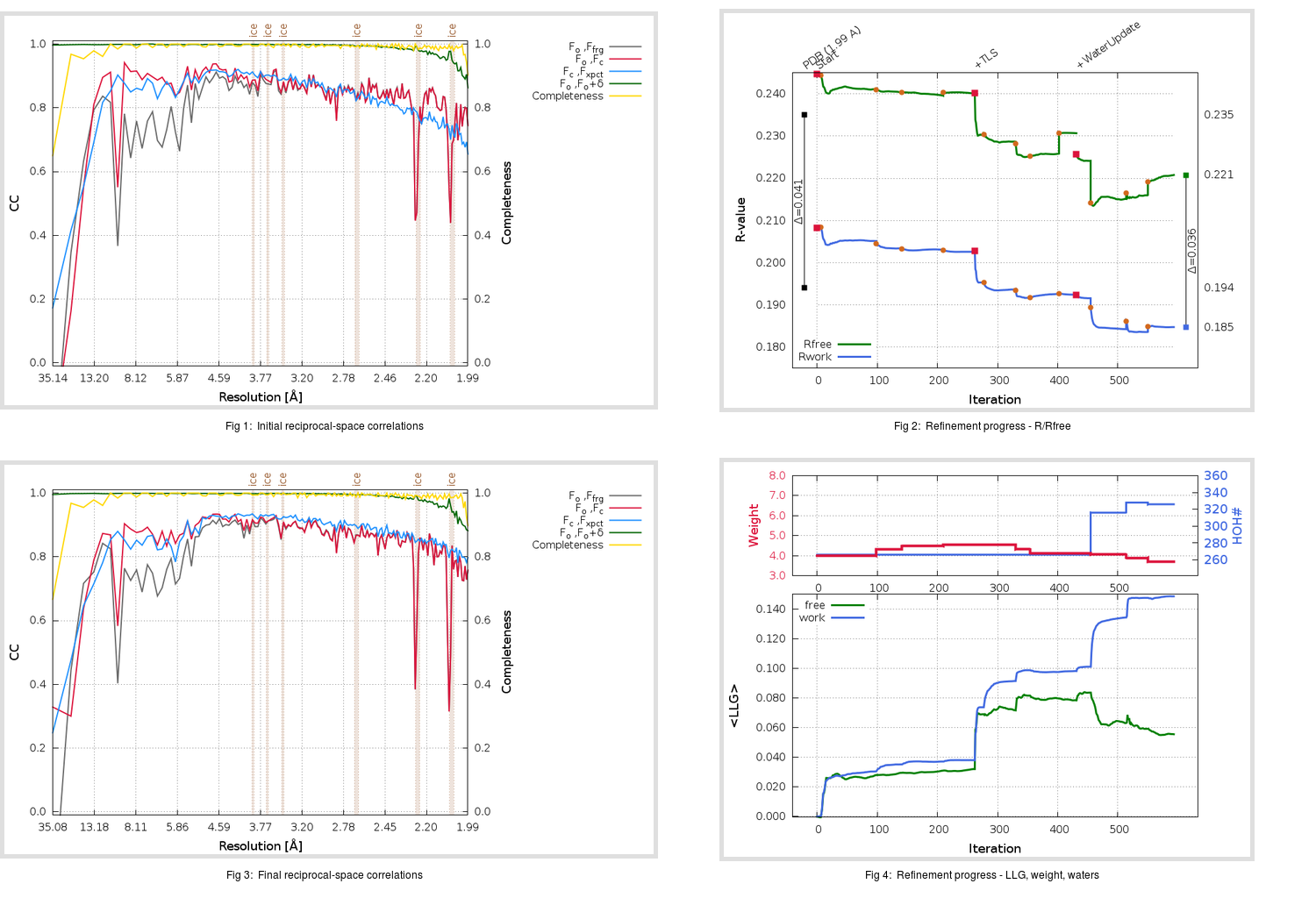Content:

```    Diffraction limits & principal axes of ellipsoid fitted to diffraction cut-off surface:
1.955         0.9571   0.0000   0.2898       a* + 0.020 c*
1.940         0.0000   1.0000   0.0000       b*
1.906        -0.2898   0.0000   0.9571      -0.505 a* + 0.863 c*
```

## Deposited

` `
 Date deposited Date data collection Resolution R, Rfree 20110821 20100806 1.99 0.1920 0.2350

Molprobity (CCP4 7.0 version) summary:

```Ramachandran outliers =   0.00 %
favored =  98.36 %
Rotamer outliers      =   1.52 %
C-beta deviations     =     1
Clashscore            =   4.19
RMS(bonds)            =   0.0156
RMS(angles)           =   1.40
MolProbity score      =   1.34
Resolution            =   1.99
R-work                =   0.1920
R-free                =   0.2350
```

```Number of waters      =   266

<B> (all atoms) =   27.84 ( sd =    9.19 ) for       2677 non-hydrogen atoms
<B>   (protein) =   27.55 ( sd =    9.00 ) for       2377 non-hydrogen atoms
<B>     (water) =   29.32 ( sd =   10.10 ) for        266 non-hydrogen atoms
<B>    (others) =   36.95 ( sd =    8.87 ) for         34 non-hydrogen atoms

B min/max       (all non-hydrogen atoms) =   10.41 /   69.61
B min/max   (protein non-hydrogen atoms) =   11.90 /   69.61
B min/max     (water non-hydrogen atoms) =   10.41 /   57.93
B min/max     (other non-hydrogen atoms) =   20.48 /   46.19
```

## BUSTER (re-)refinement

` `

Molprobity (CCP4 7.0 version) summary:

```Ramachandran outliers =   0.00 %
favored =  97.70 %
Rotamer outliers      =   1.14 %
C-beta deviations     =     0
Clashscore            =   2.09
RMS(bonds)            =   0.0117
RMS(angles)           =   1.53
MolProbity score      =   1.09
Resolution            =   1.99
R-work                =   0.1847
R-free                =   0.2208
```

```Number of waters      =   326

<B> (all atoms) =   30.18 ( sd =   10.73 ) for       2737 non-hydrogen atoms
<B>   (protein) =   28.40 ( sd =    9.32 ) for       2377 non-hydrogen atoms
<B>     (water) =   42.24 ( sd =   12.38 ) for        326 non-hydrogen atoms
<B>    (others) =   39.20 ( sd =    5.74 ) for         34 non-hydrogen atoms

B min/max       (all non-hydrogen atoms) =   13.19 /   80.90
B min/max   (protein non-hydrogen atoms) =   14.27 /   77.13
B min/max     (water non-hydrogen atoms) =   13.19 /   80.90
B min/max     (other non-hydrogen atoms) =   29.09 /   45.52
```

Refinement progression:Results:

` `
 File Remark 3TIT_aB_refine.01_03_refine.pdb.gz exact refinement commands are in header 3TIT_aB_refine.01_03_refine.mtz.gz including original deposited data and several re-refinement map coefficients 3TIT_aB_refine.01_03_BUSTER_model.cif.gz including any non-standard compound restraints 3TIT_aB_refine.01_03_BUSTER_refln.cif.gz